# SSC Quantitative Aptitude Questions (Day-80)

Dear Aspirants, you can find the Quantitative Aptitude questions with detailed explanations for the SSC exams. Nowadays the competitive level of the exam has been increasing consistently. Due to the great demand for the government job, the level of the toughness reached greater. Candidates have to enhance the preparation process in order to drive in the right path. It doesn’t need to clear the prescribed cutoff. You must have to score good marks more than the cut off marks to get into the final provisional list. Here we have updating the Quantitative Aptitude questions with detailed explanations on a daily basis. You can practice with us and measure your level of preparation. According to that you can sculpt yourself in a proper way. SSC aspirants kindly make use of it and grab your success in your career.

Start Quiz

1) A sum of Rs 3100 is lent out at simple interest in two parts. One at 8% per annum and another at 6% per annum. If the total annual interest is Rs 212,then what is the money (in Rs) lent at rate of 8%?

(a) 1000

(b) 1250

(c) 1300

(d) 1400

Direction (2-5): The table given below shows the percentage of people reading various newspapers in a society. It also shows the ratio of males and females for each newspaper. Each person reads exactly 1 newspaper. Total number of people in society is 20000.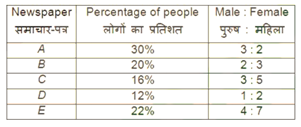2) How many females read newspaper A?

(a) 3600

(b) 3200

(c) 2400

(d) 2800

3) What is difference in number of males reading newspaper B and number of males reading newspaper D?

(a) 1600

(b) 2000

(c) 2400

(d) 800

4) For which two newspaper the number of females reading them is same?

(a) D and E

(b) C and E

(c) A and B

(d) A and C

5) By how much percent females reading newspaper E are more than the males reading newspaper C?

(a) 233.33

(b) 133.33

(c) 266.66

(d) 333.33

6) If length and breadth of a cuboid is increased by 20%, then by how much percent the height should be reduced to keep the volume same?

(a) 40

(b) 44

(c) 30 5/9

(d) 33 4/7

7) The volume of a conical tent is 154 cm3 and the area of its base is 38.5 cm2. What is the length (in cm) of canvas required to build the tent, if thecanvas is 2 cm in width?

154338.522,

(a) 71.35

(b) 68.75

(c) 73.25

(d) 75.75

8) A metallic sphere is melted and moulded to form conical shaped bullets. If radius of the bullet is a twice of its height and radius of bullet is half of theradius of the metallic sphere, then what is the numbers of bullet formed?

(a) 32

(b) 16

(c) 128

(d) 64

9) A chord of length 24 cm is drawn in a circle of diameter 40 cm. Another chord of length 32 cm is drawn in the same circle parallel to 24 cm long chord.What is the minimum distance (in cm) between them?

(a) 4

(b) 2

(c) 8

(d) 3

10) If the length of a rectangle is increased by 50%, then by how much percent its breadth should be reduced to keep the area the same?

(a) 50

(b) 33 1/3

(c) 66 1/6

(d) 37 1/6

At 8% rate interest will be = 248 Rs.

At 6% rate interest amount = 186 Rs.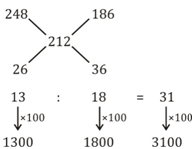% of people who read newspaper

= 30 × 2/5

= 12%

Total number of female who read newspaper A

= 12/100 × 20000

= 2400

Males reading newspaper B in terms of total people

= 20/1 × 2/5

= 8%

Male reading newspaper D in terms of % of total people

= 12 × 1/3

= 4%

Difference = (8 – 4)% ⇒ 4% of total people

⇒ 4/100 × 20000

= 800

No. of female in terms of % of total no. of people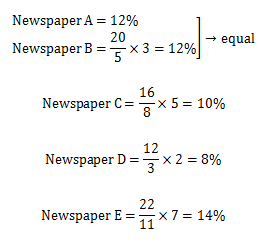= 16/8 × 3 = 6% of total

Females reading newspaper E = 14%

Hence

⇒ (14 – 6) / 6 ⇒ 133.33

ℓbh = 12ℓ × 1.2b × h’

h / 1.44 = h^’

0.69444h = h’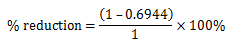=30.55%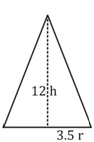Area of base of cone = 38.5 = πr²

r = 3.5 cm

Vol. cone ⇒ ⅓ πr²h

154 = ⅓× 38.5h

h = 12 cm

ℓ = √(h2 + r2 )

ℓ = 12.5 cm

C.S.A. of cone = πr ℓ

= 22/7 × 3.5 × 12.5

= 11 × 12.5

= 137.5

If 2 cm is width of the canvas then total length required

= 137.5/2 = 68.75 cm

Vol. of sphere = 4/5 πr³

Radius of conical bullet = r’ = r/2

Height of conical bullet = h = r/2 = r/4

No. of bullets = n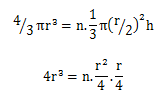n = 64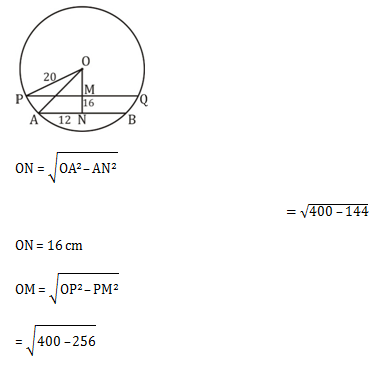= 12 cm

Min difference between these two chord = 16 – 12 = 4cm

ℓb = 15ℓb’

.66b = b’

Reduction in the breadth is 33.33%

 Check Here to View SSC CGL / CHSL 2021 Quantitative Aptitude Questions Day – 79 Day – 78 Day – 77